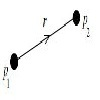Search by Topic

Resources tagged with Physics similar to Stereoisomers:

Filter by: Content type:
Age range:
Challenge level:

There are 77 results

Broad Topics > Applications > Physics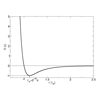Lennard Jones Potential

Age 16 to 18 Challenge Level:

Investigate why the Lennard-Jones potential gives a good approximate explanation for the behaviour of atoms at close rangesStriking Gold

Age 16 to 18 Challenge Level:

Investigate some of the issues raised by Geiger and Marsden's famous scattering experiment in which they fired alpha particles at a sheet of gold.Constantly Changing

Age 14 to 16 Challenge Level:

Many physical constants are only known to a certain accuracy. Explore the numerical error bounds in the mass of water and its constituents.Big and Small Numbers in Chemistry

Age 14 to 16 Challenge Level:

Get some practice using big and small numbers in chemistry.The Amazing Properties of Water

Age 14 to 18 Challenge Level:

Find out why water is one of the most amazing compounds in the universe and why it is essential for life. - UNDER DEVELOPMENTDiamonds Aren't Forever

Age 16 to 18 Challenge Level:

Ever wondered what it would be like to vaporise a diamond? Find out inside...Eudiometry

Age 16 to 18 Challenge Level:

When a mixture of gases burn, will the volume change?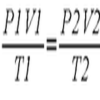Ideal Axes

Age 16 to 18 Challenge Level:

Explore how can changing the axes for a plot of an equation can lead to different shaped graphs emergingThe Power of Dimensional Analysis

Age 14 to 18

An introduction to a useful tool to check the validity of an equation.Motorbike Momentum

Age 16 to 18 Challenge Level:

A think about the physics of a motorbike riding upside down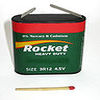Battery Modelling

Age 16 to 18 Challenge Level:

Find out how to model a battery mathematicallyCobalt Decay

Age 16 to 18 Challenge Level:

Investigate the effects of the half-lifes of the isotopes of cobalt on the mass of a mystery lump of the element.The Real Hydrogen Atom

Age 16 to 18 Challenge Level:

Dip your toe into the world of quantum mechanics by looking at the Schrodinger equation for hydrogen atomsMaths in the Undergraduate Physical Sciences

Age 16 to 18

An article about the kind of maths a first year undergraduate in physics, engineering and other physical sciences courses might encounter. The aim is to highlight the link between particular maths. . . .Physnrich

Age 14 to 18 Challenge Level:

PhysNRICH is the area of the StemNRICH site devoted to the mathematics underlying the study of physicsCore Scientific Mathematics

Age 14 to 18 Challenge Level:

This is the area of the advanced stemNRICH site devoted to the core applied mathematics underlying the sciences.Gravity Paths

Age 16 to 18 Challenge Level:

Where will the spaceman go when he falls through these strange planetary systems?Ideal Gases

Age 16 to 18 Challenge Level:

Problems which make you think about the kinetic ideas underlying the ideal gas laws.Construct the Solar System

Age 14 to 18 Challenge Level:

Make an accurate diagram of the solar system and explore the concept of a grand conjunction.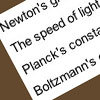Universal Time, Mass, Length

Age 16 to 18 Short Challenge Level:

Can you work out the natural time scale for the universe?Perfect Eclipse

Age 14 to 16 Challenge Level:

Use trigonometry to determine whether solar eclipses on earth can be perfect.The Lorentz Force Law

Age 16 to 18 Challenge Level:

Explore the Lorentz force law for charges moving in different ways.Pack Man

Age 16 to 18 Challenge Level:

A look at different crystal lattice structures, and how they relate to structural propertiesApproximately Certain

Age 14 to 18 Challenge Level:

Estimate these curious quantities sufficiently accurately that you can rank them in order of sizeNew Units for Old

Age 16 to 18 Challenge Level:

Can you match up the entries from this table of units?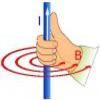Electromagnetism

Age 16 to 18

Read all about electromagnetism in our interactive article.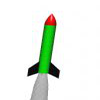Go Spaceship Go

Age 16 to 18 Challenge Level:

Show that even a very powerful spaceship would eventually run out of overtaking powerDrug Stabiliser

Age 16 to 18 Challenge Level:

How does the half-life of a drug affect the build up of medication in the body over time?The Ultra Particle

Age 16 to 18 Challenge Level:

Explore the energy of this incredibly energetic particle which struck Earth on October 15th 1991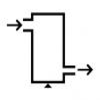Keep Your Momentum Going

Age 16 to 18 Challenge Level:

A look at a fluid mechanics technique called the Steady Flow Momentum Equation.Levels of Bohr

Age 16 to 18 Challenge Level:

Look at the units in the expression for the energy levels of the electrons in a hydrogen atom according to the Bohr model.Big and Small Numbers in Physics

Age 14 to 16 Challenge Level:

Work out the numerical values for these physical quantities.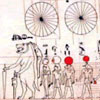Ancient Astronomical Terms

Age 11 to 18

Some explanations of basic terms and some phenomena discovered by ancient astronomersPumping the Power

Age 16 to 18 Challenge Level:

What is an AC voltage? How much power does an AC power source supply?Mach Attack

Age 16 to 18 Challenge Level:

Have you got the Mach knack? Discover the mathematics behind exceeding the sound barrier.Earth Orbit

Age 16 to 18 Challenge Level:

Follow in the steps of Newton and find the path that the earth follows around the sun.Moving Stonehenge

Age 16 to 18 Challenge Level:

A look at the fluid mechanics questions that are raised by the Stonehenge 'bluestones'.The Not-so-simple Pendulum 1

Age 16 to 18 Challenge Level:

See how the motion of the simple pendulum is not-so-simple after all.Cannon Balls

Age 16 to 18 Short Challenge Level:

How high will a ball taking a million seconds to fall travel?Escape from Planet Earth

Age 16 to 18 Challenge Level:

How fast would you have to throw a ball upwards so that it would never land?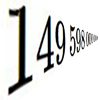Big and Small Numbers in Physics - Group Task

Age 16 to 18 Challenge Level:

Work in groups to try to create the best approximations to these physical quantities.Resistance

Age 16 to 18 Challenge Level:

Find the equation from which to calculate the resistance of an infinite network of resistances.Sweeping Satellite

Age 16 to 18 Challenge Level:

Derive an equation which describes satellite dynamics.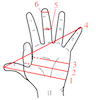Age 14 to 16 Challenge Level:

Which units would you choose best to fit these situations?A Question of Scale

Age 14 to 16 Challenge Level:

Use your skill and knowledge to place various scientific lengths in order of size. Can you judge the length of objects with sizes ranging from 1 Angstrom to 1 million km with no wrong attempts?Whose Line Graph Is it Anyway?

Age 16 to 18 Challenge Level:

Which line graph, equations and physical processes go together?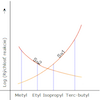Reaction Types

Age 16 to 18 Challenge Level:

Explore the rates of growth of the sorts of simple polynomials often used in mathematical modelling.Powerfully Fast

Age 16 to 18 Challenge Level:

Explore the power of aeroplanes, spaceships and horses.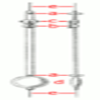The Not-so-simple Pendulum 2

Age 16 to 18 Challenge Level:

Things are roughened up and friction is now added to the approximate simple pendulum